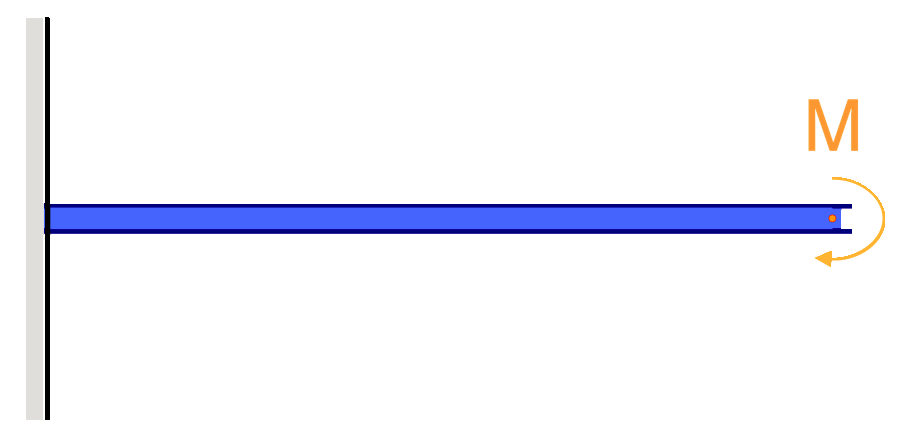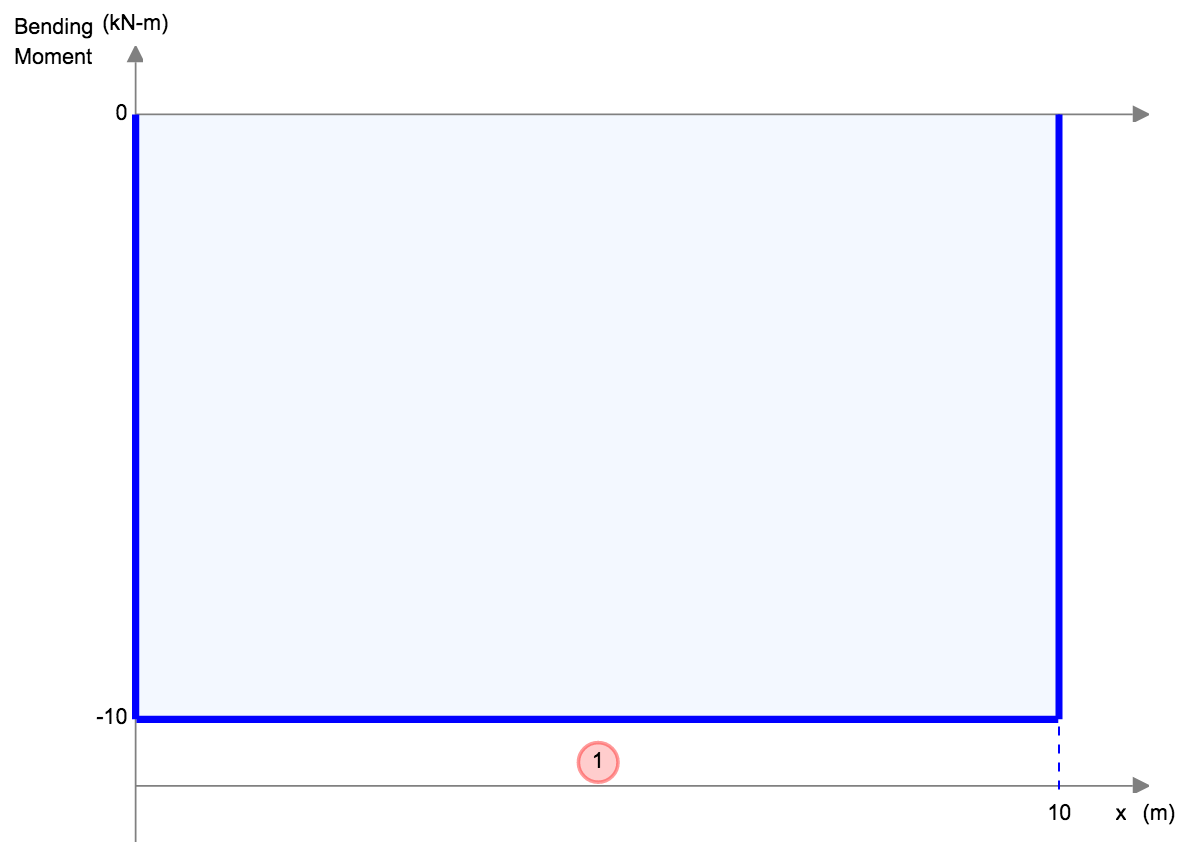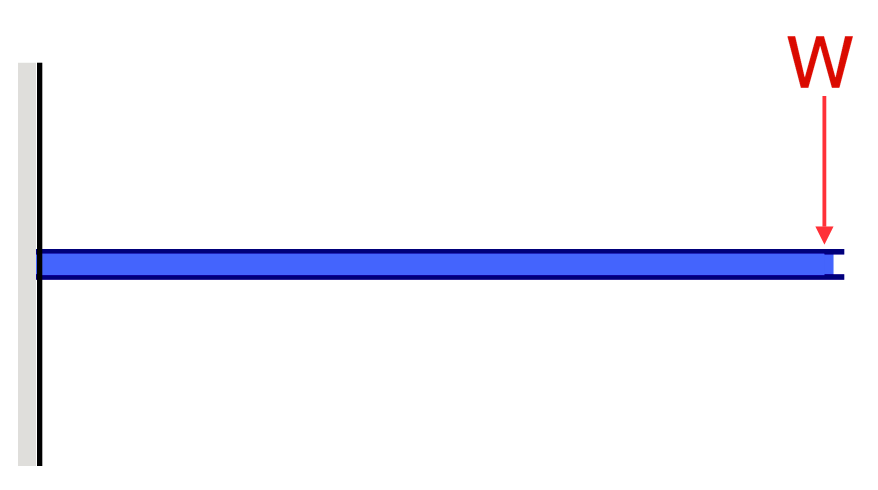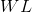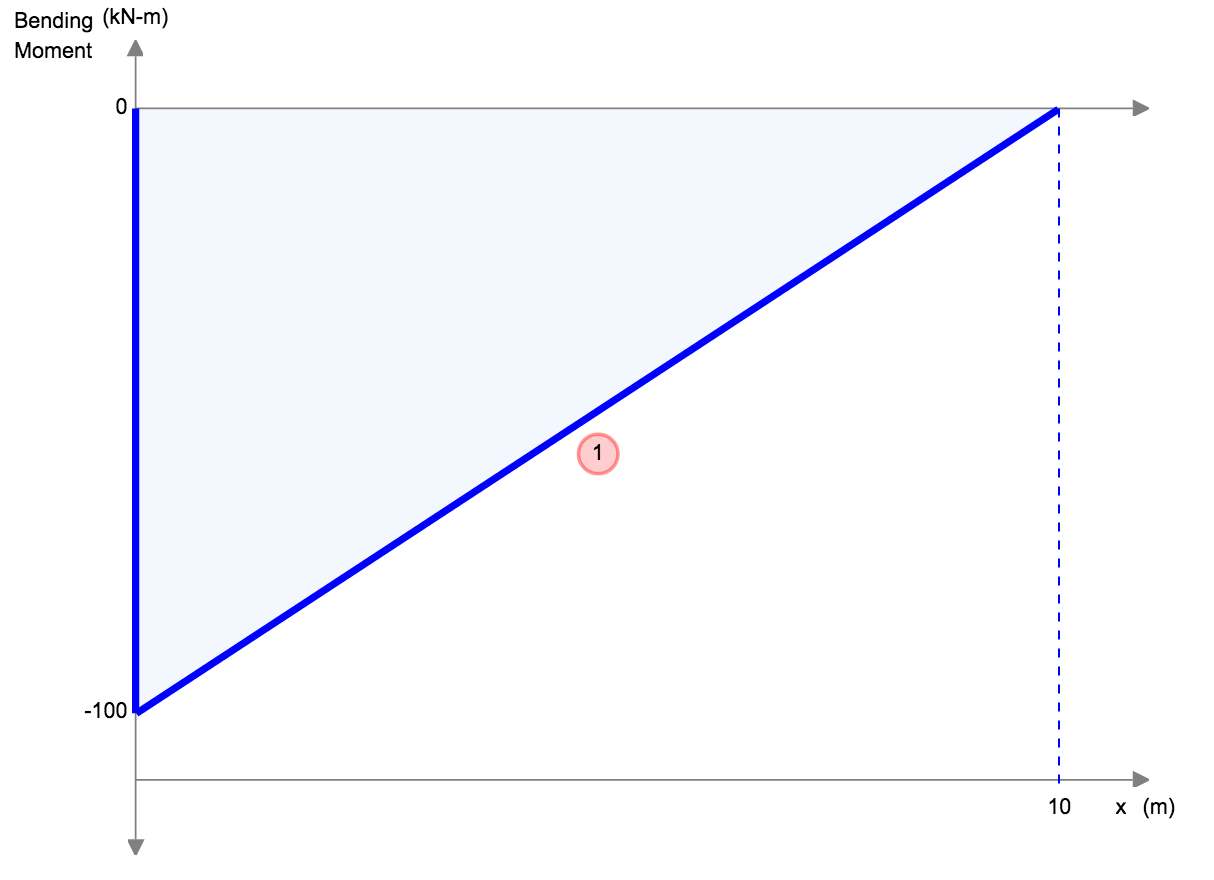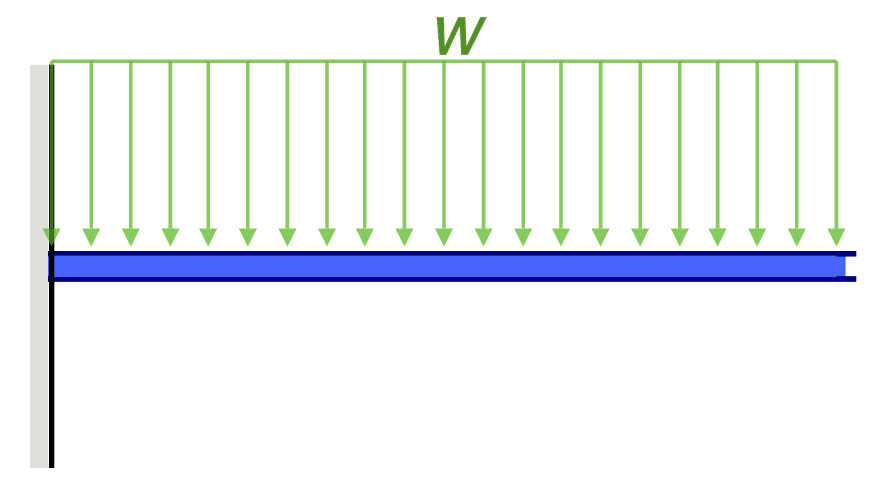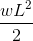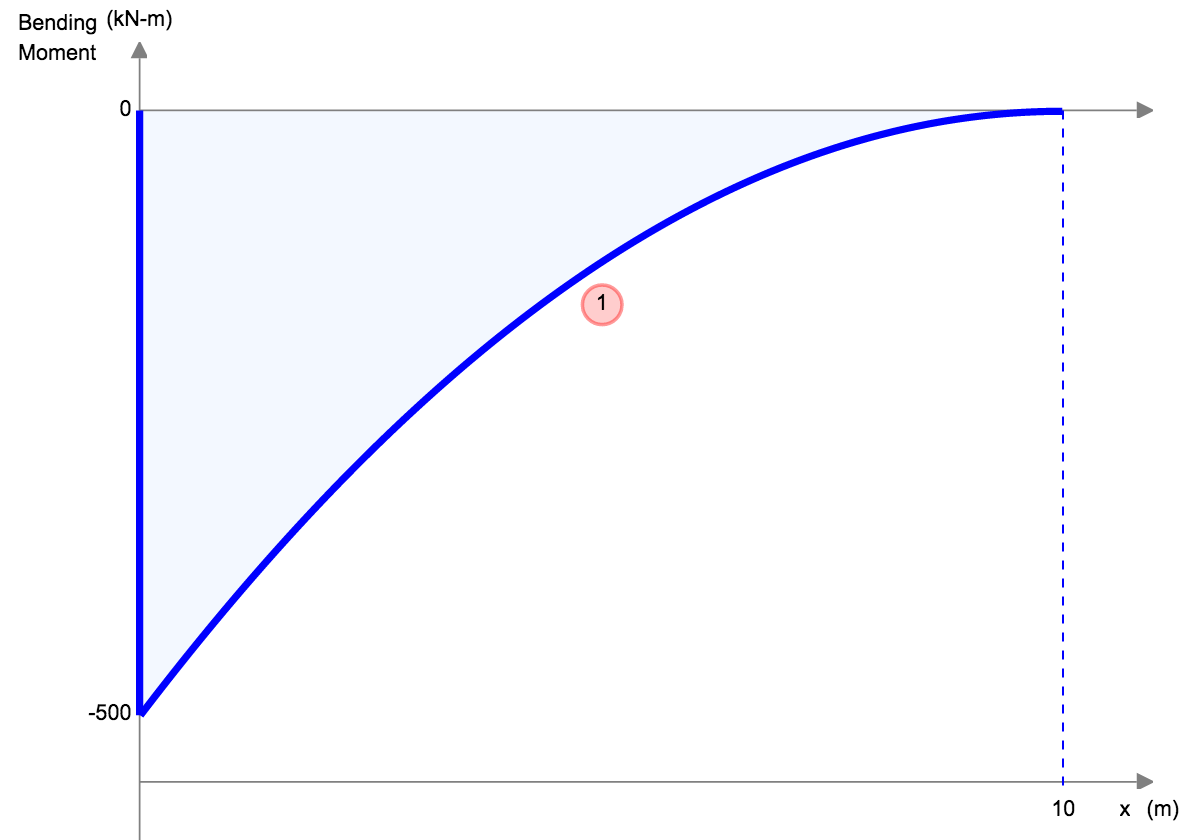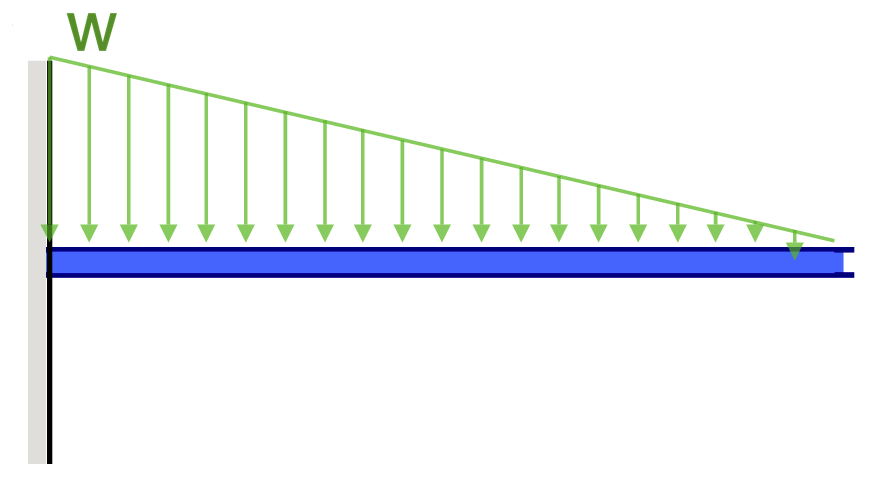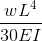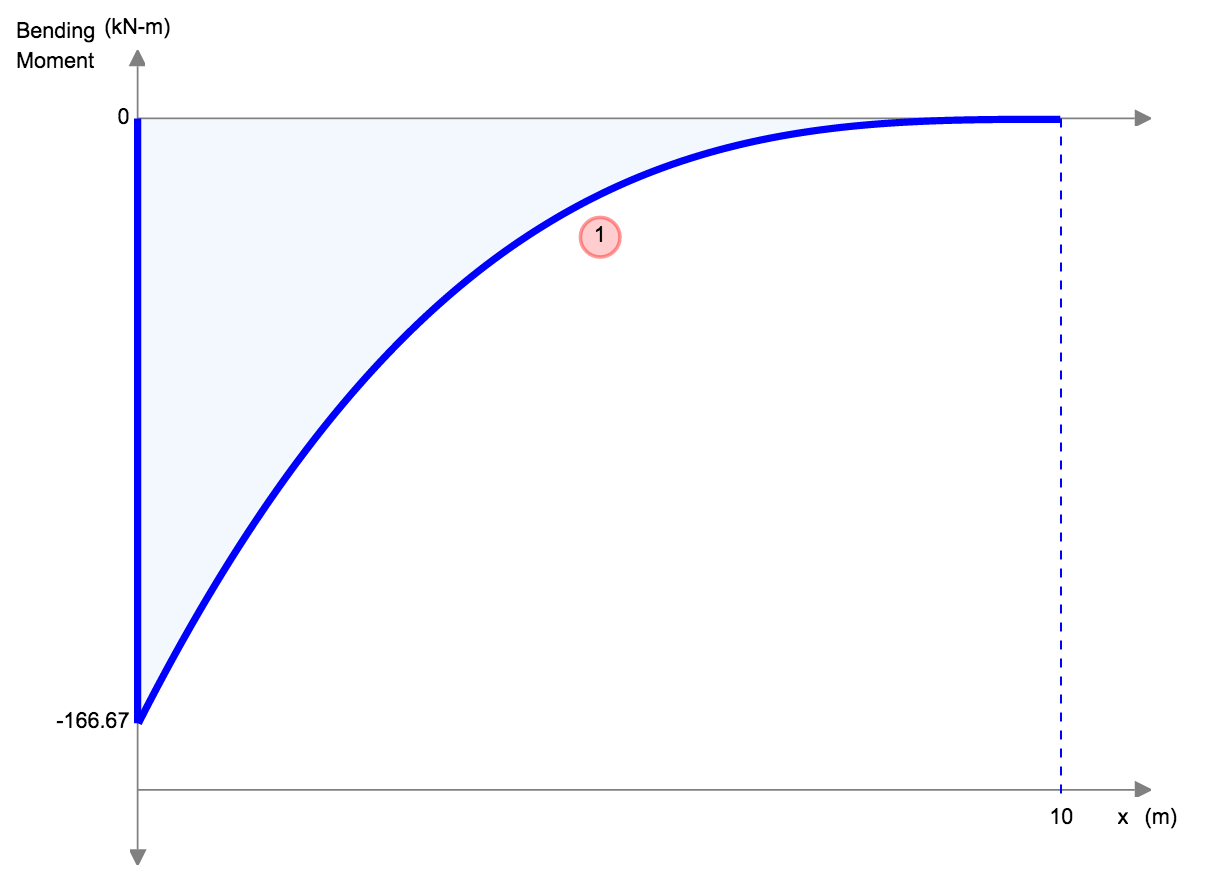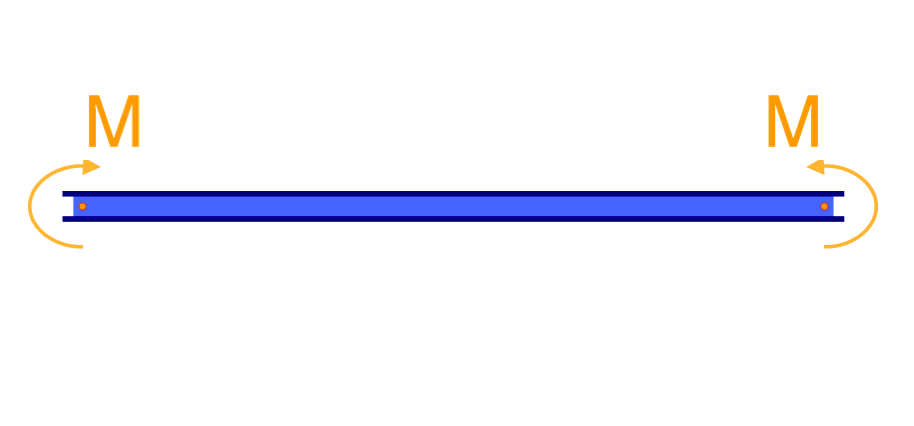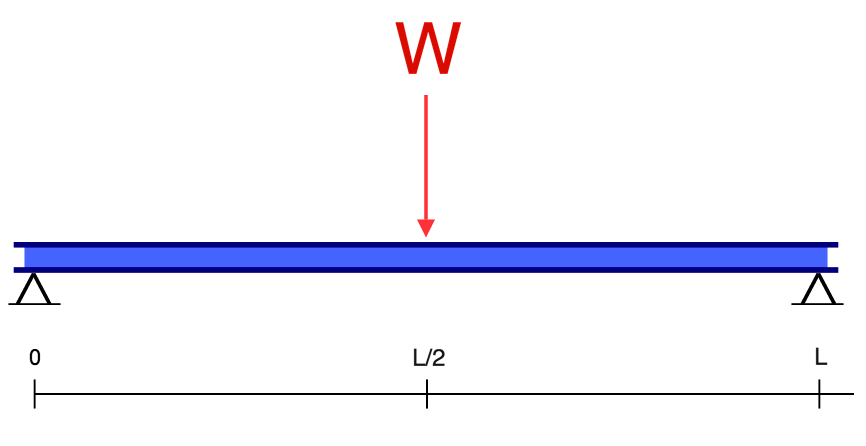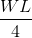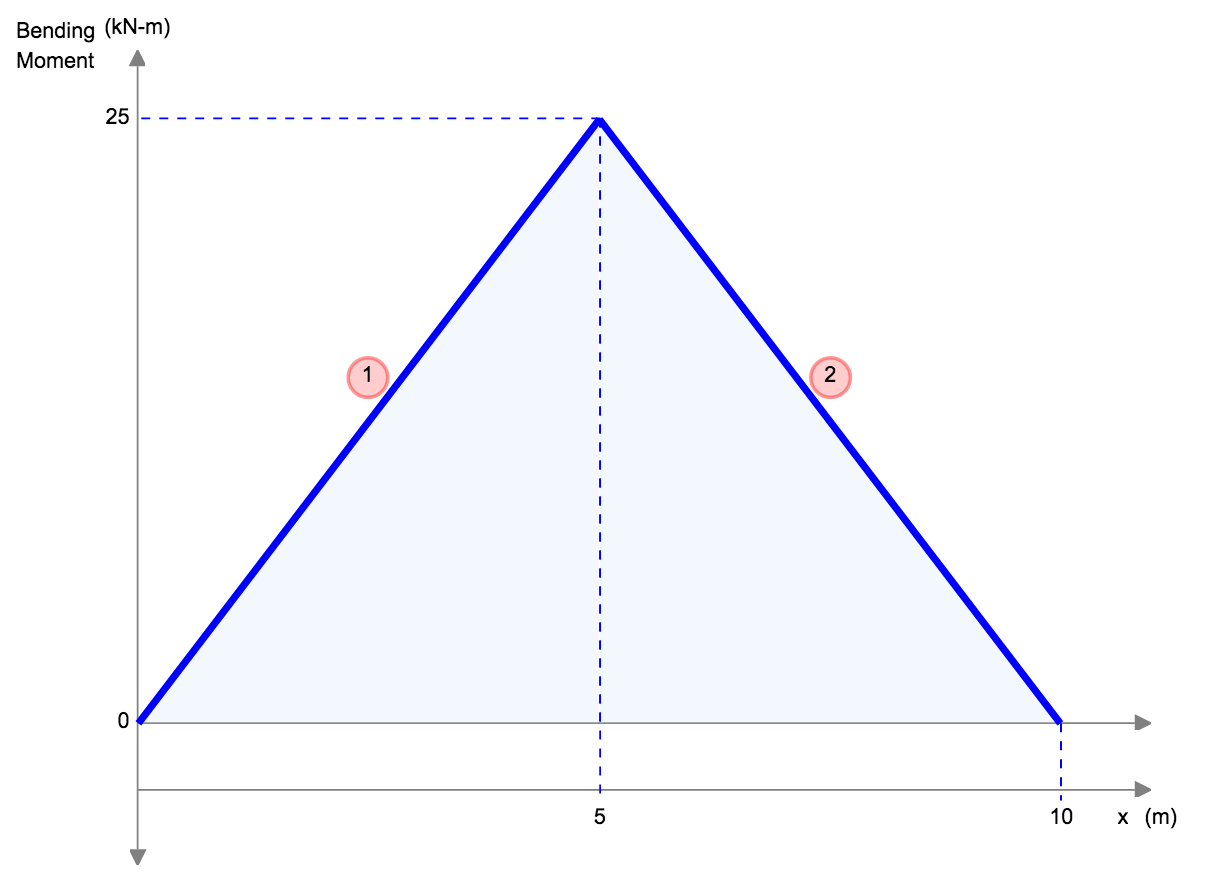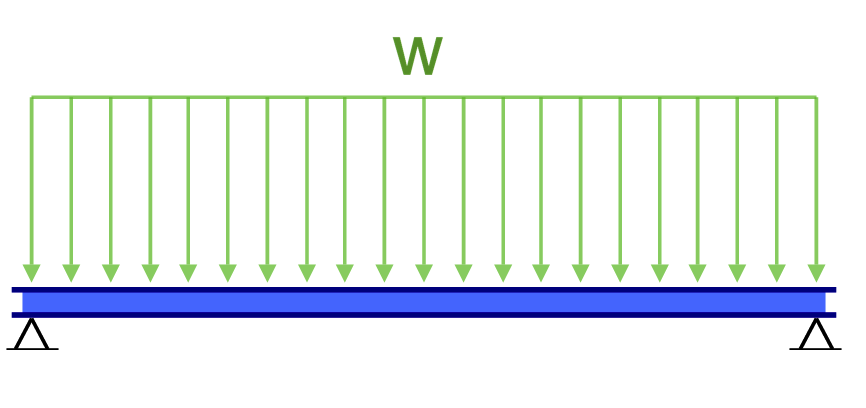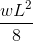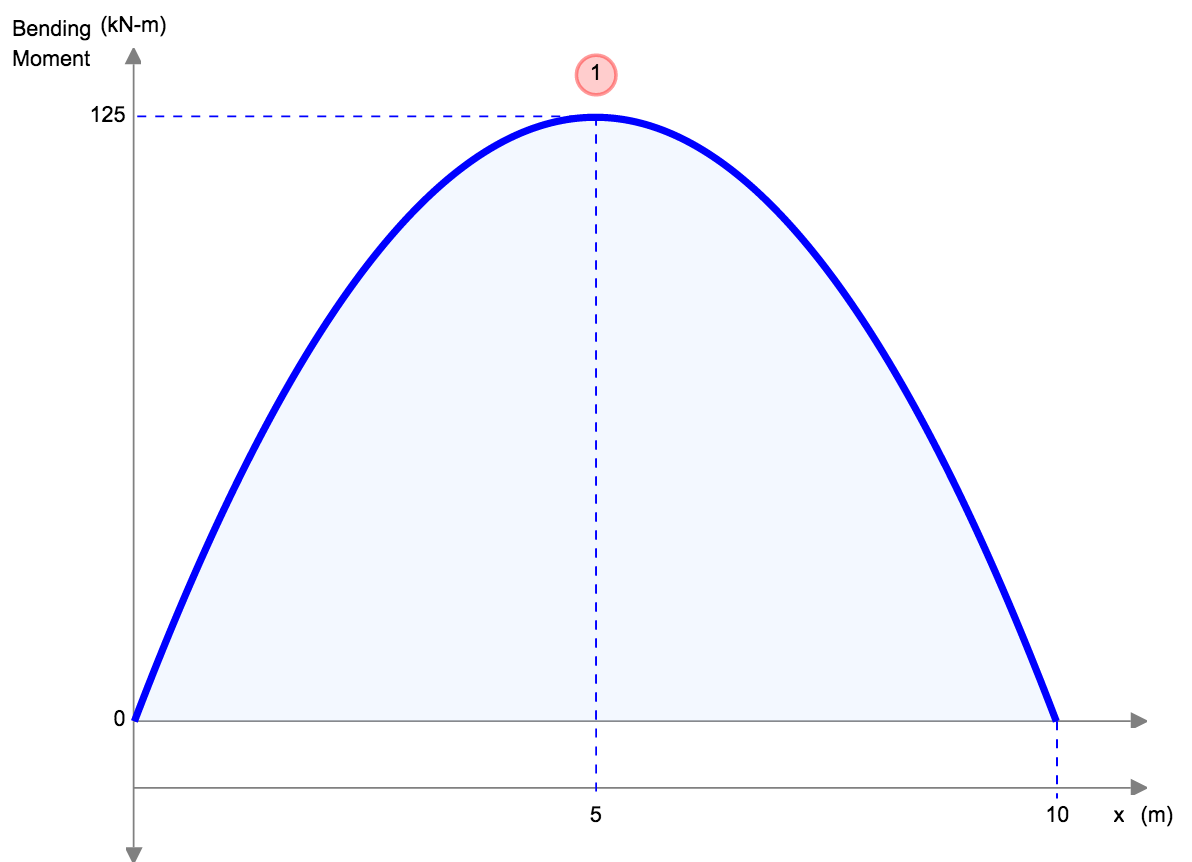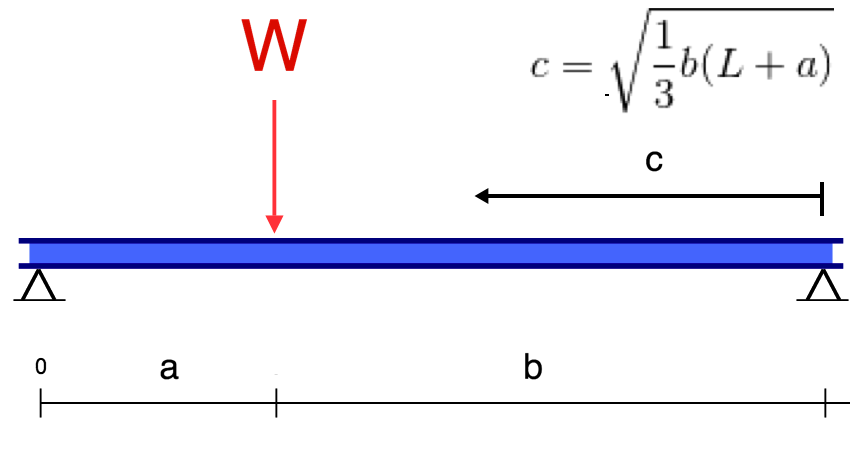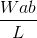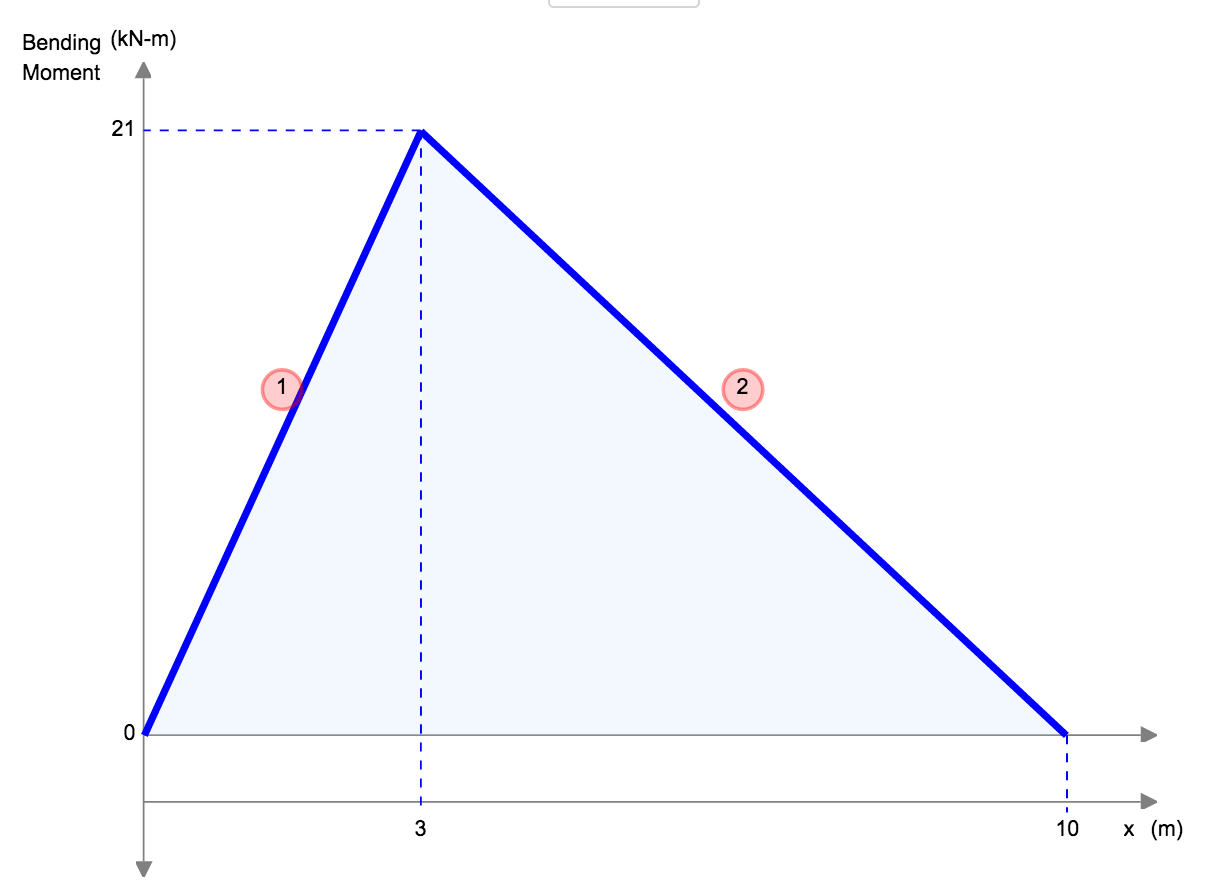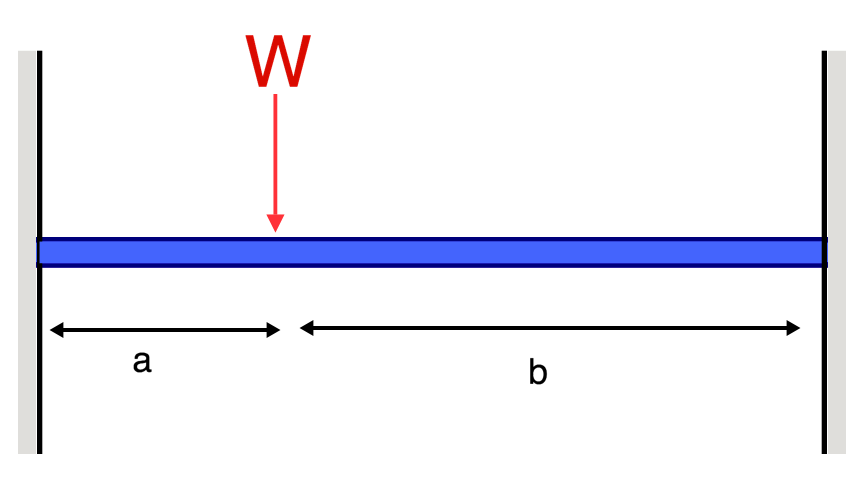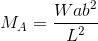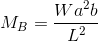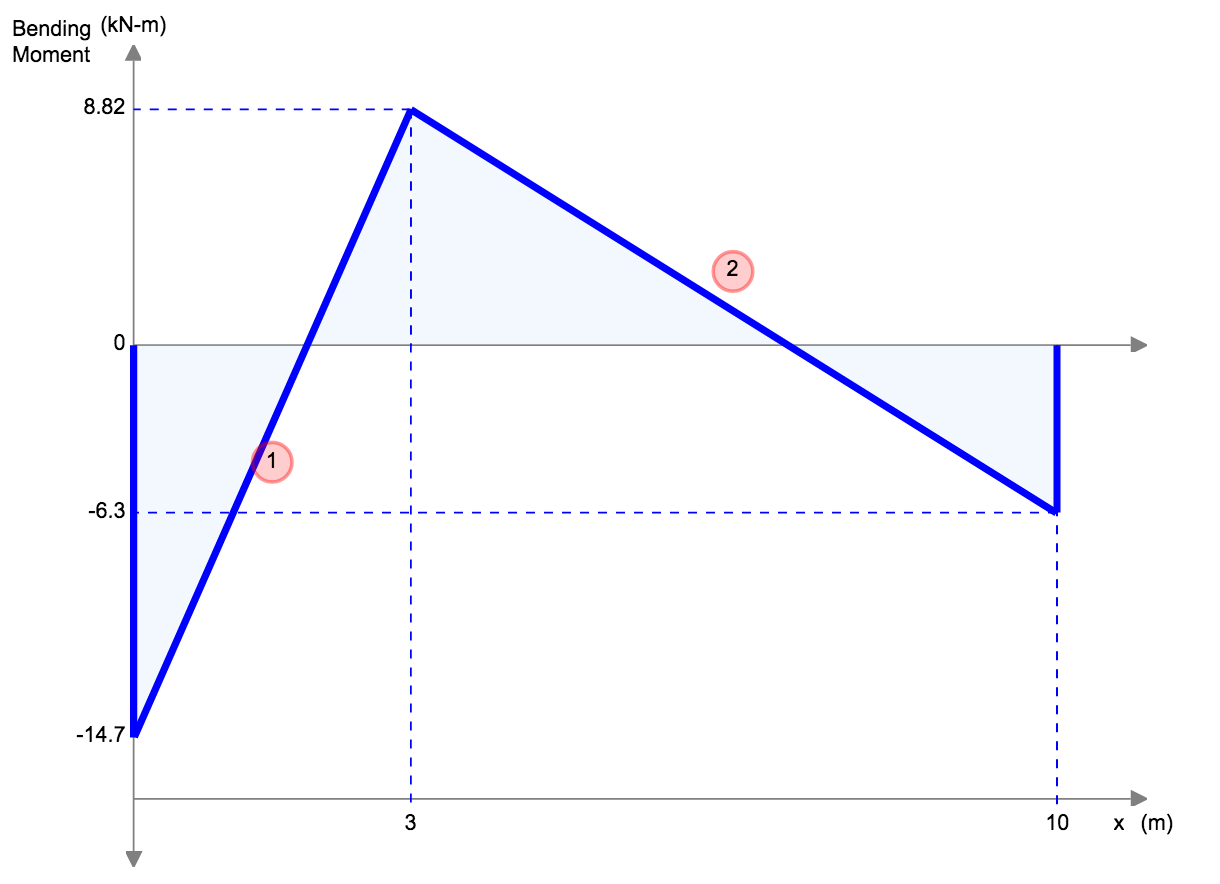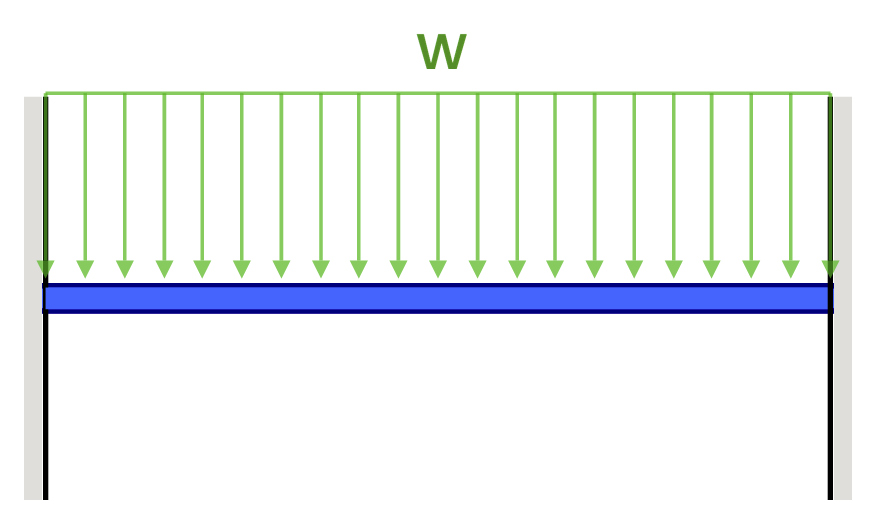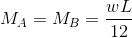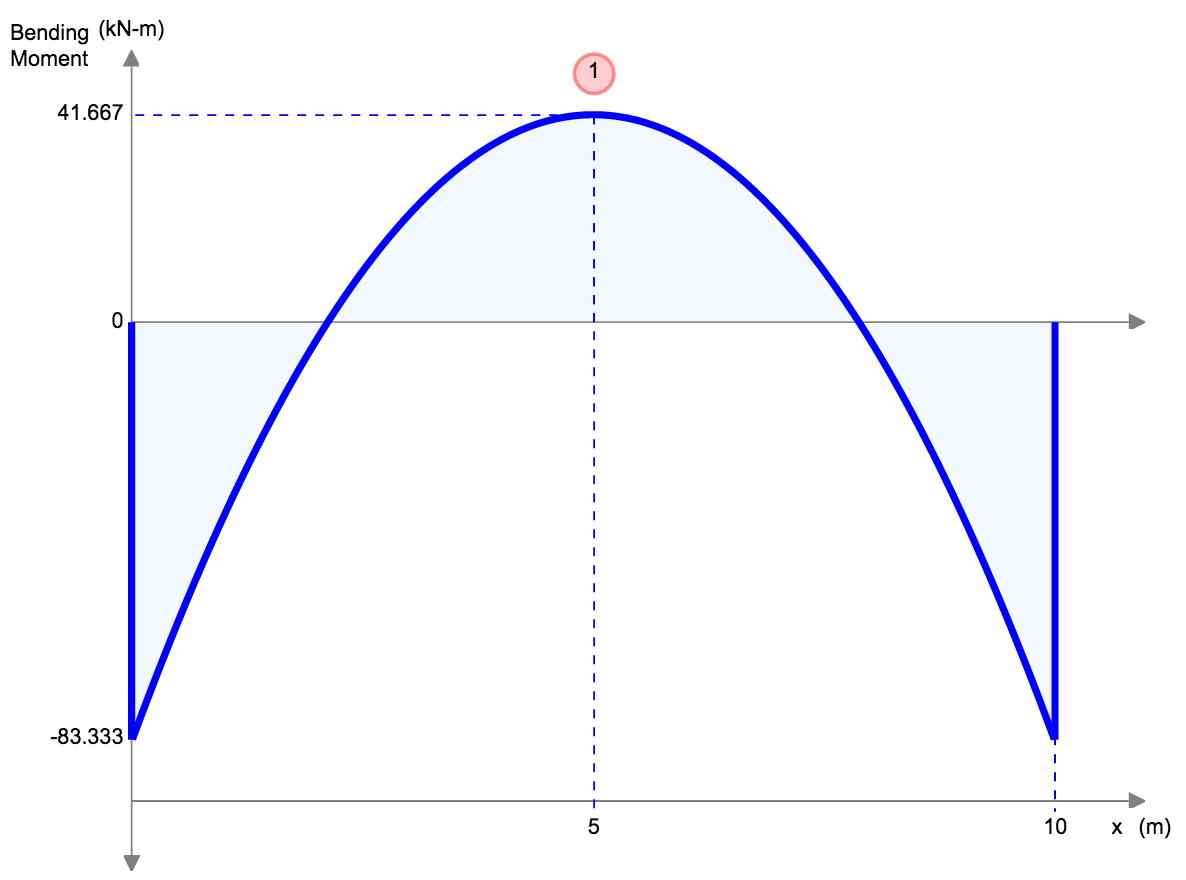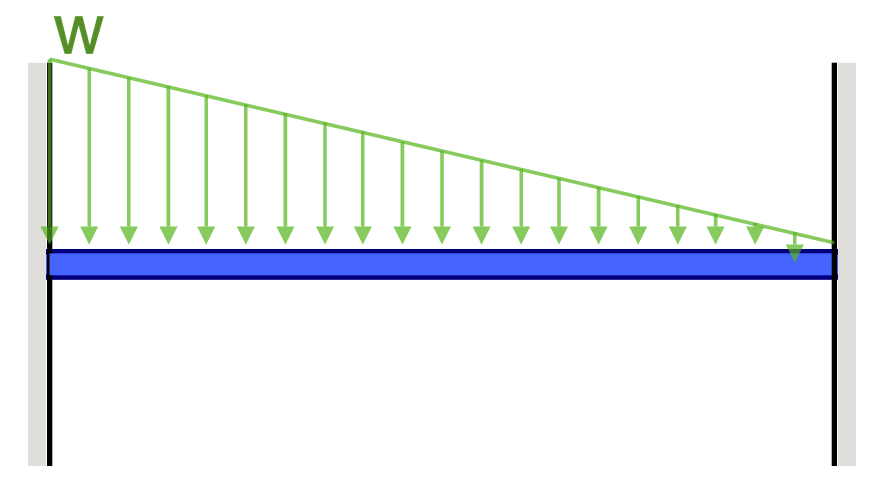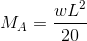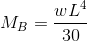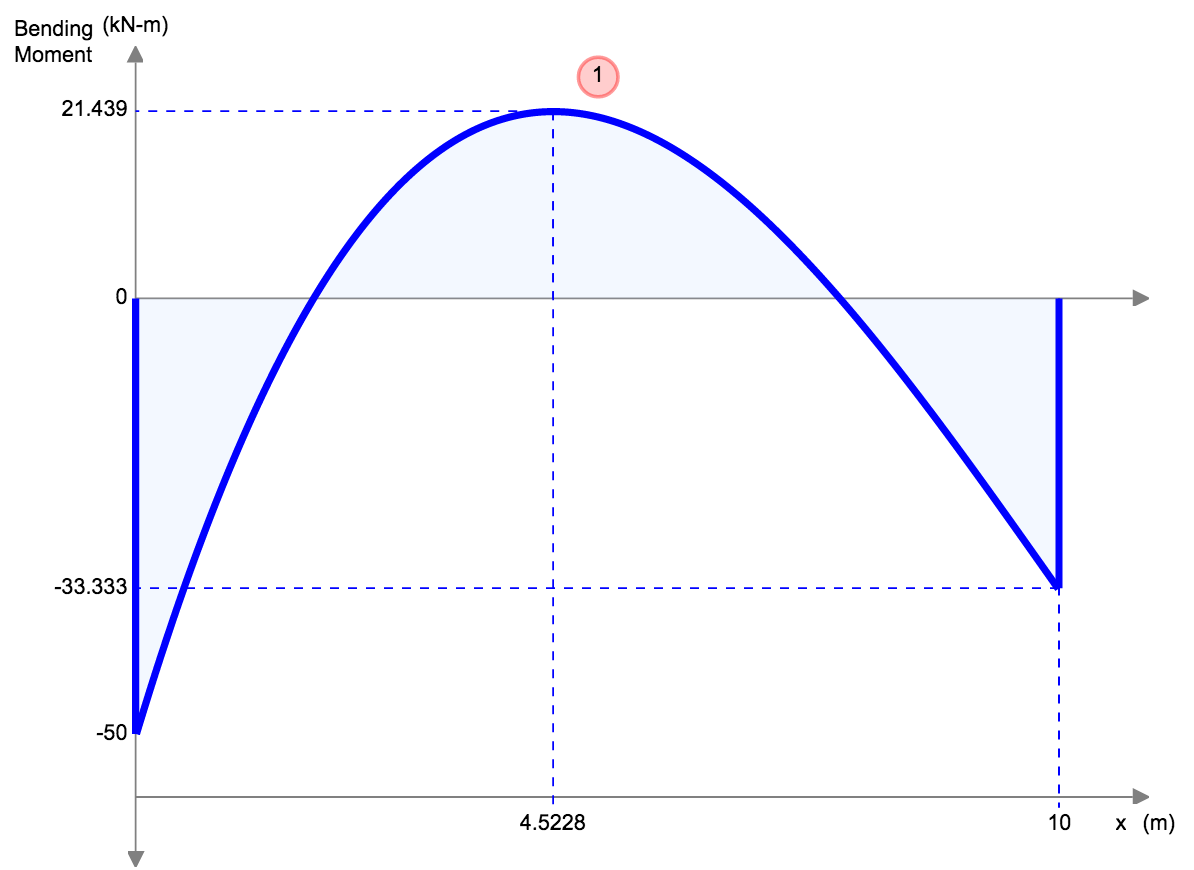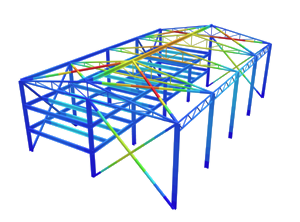SkyCiv Documentation

Your guide to SkyCiv software - tutorials, how-to guides and technical articles

1. Home
2. Tutorials
3. Equations and Summaries
4. Bending Moment Equations

# Bending Moment Equations

## Bending Moment Equations for Beams

Bending Moment Equations offer a quick and easy analysis to determine the maximum bending moment in a beam. Below is a concise table that shows the bending moment equations for different beam setups. Don’t want to hand calculate these, sign up for a free SkyCiv Account and get instant access to a free version of our beam software!

Use the equations and formulas below to calculate the max bending moment in beams. Click the ‘check answer’ button to open up our free beam calculator. Bending moment equations are perfect for quick hand calculations and quick designs.

Find what you’re looking for faster:

## Bending Moment Equations Table

Reference Max Bending Moment Shape